# Abstract

Recent research works demonstrated that the interaction between the loads and the carrying structure's boundary which is related to the inertia of the load is an influential factor on the dynamic response of the structure. Although effects of the inertia in moving loads were considered in many works, very few papers can be found on the inertial effects of the stationary loads on structures. In this paper, an elastodynamic formulation was employed to investigate the dynamic response of a homogeneous isotropic elastic half-space under an inertial strip foundation subjected to a time-harmonic force. Fourier integral transformation was used to solve the system of Poisson-type partial differential equation considering the boundary conditions and the inertial effects. Steepest descent method was employed to obtain the approximate far-field displacements and stresses. A numerical example is presented to illustrate the methodology and typical results.

Inertial Foundation; Poisson-type partial differential equation; Wave Propagation; Elasticity

Far-field dynamic behavior of a half-space under an inertial strip foundation subjected to a time-harmonic force

M. DehestaniI, * * Author email: dehestani@gmail.com ; N. R. MalidarrehII; A. J. ChoobbastiIII; A. VafaiIV

IAssistant Professor, Faculty of Civil Engineer- ing, Babol University of Technology, Babol, Postal Box: 484, Babol, Postal Code: 47148- 71167, I.R. Iran Tel: +981113231707 Fax: +981113231707

IIIAssociate Professor, Dept. of Civil Engineering, Babol University of Technology, Babol, Iran

IVProfessor, Dept. of Civil Engineering, Sharif University of Technology, Tehran, Iran

ABSTRACT

Recent research works demonstrated that the interaction between the loads and the carrying structure's boundary which is related to the inertia of the load is an influential factor on the dynamic response of the structure. Although effects of the inertia in moving loads were considered in many works, very few papers can be found on the inertial effects of the stationary loads on structures. In this paper, an elastodynamic formulation was employed to investigate the dynamic response of a homogeneous isotropic elastic half-space under an inertial strip foundation subjected to a time-harmonic force. Fourier integral transformation was used to solve the system of Poisson-type partial differential equation considering the boundary conditions and the inertial effects. Steepest descent method was employed to obtain the approximate far-field displacements and stresses. A numerical example is presented to illustrate the methodology and typical results.

Keywords: Inertial Foundation; Poisson-type partial differential equation; Wave Propagation; Elasticity

1. Introduction

Understanding the dynamic response of half-space subjected to different surface loads is of considerable practical interest for scientists and engineers. Dynamic response of a half-space subjected to concentrated loads at the surface or inside the half-space which was investigated by Horace Lamb  is the first well-known work in this field. The use of complex contour integration in this work yielded the wave motions generated at the surface of the elastic half-space. Following study in this area is the work of Miller and Pursey , in which Lamb's method was changed to provide definite integral representations of the field at an arbitrary point in an isotropic half-space due to the surface tractions. Asymptotic expansions were obtained and the results for the displacement components were achieved by polar diagrams. Finally the radiation impedances were acquired by numerical procedures for a number of cases.

Due to the practical importance of the research in this area, a great number of research works have been accomplished on the response of half-space subjected to different types of loads. Guangyu and Kaixin  studied a two-dimensional Lamb's problem subjected to an impact loading prescribed on a finite strip area of the surface. An exact algebraic solution was obtained by employing the integral transformation method and utilizing an inversion technique. The results were used to study the excitation and propagation processes of stress waves, including the longitudinal wave, the transverse wave and Rayleigh-wave.

Dynamic stresses of a half-space subjected to a moving wheel-type load prescribed on a finite patch were investigated by Dehestani et al . In their paper, influences of the different types of load transfer mechanisms from the moving object to half-space have been studied.

The main theoretical aspects of analysis of moving loads in a local coordinate frame were studied in a paper by Andersen et al . They discussed the steps in the finite-element and boundary element method formulations. Also, the problems in describing material dissipation in the moving frame of reference were addressed. Finally, a methodology for the coupling of a local finite element model with a boundary-element model of an exterior, or open, domain was illustrated.

For moving loads on simple structures, the inertial influences of the loads have been investigated in many papers e.g. [6-9]. Moving loads with inertial effects are known as moving masses in this area. Final results in these papers revealed that the influence of the inertia of the moving object in some cases can affect the dynamic response of the structure significantly. Dynamic responses of structures under moving loads were addressed in a comprehensive book by Fryba  in which the problem was considered in vast applicable conditions.

Although there are several works on moving inertial loads (masses) on structures, very few studies were reported on stationary inertial foundation subjected to dynamic load. Rades  introduced a Kerr-type inertial foundation model in order to obtain the approximate dynamic response of a particular kind of vibration isolator. The analytical model was established upon the simplest 2D problem regarding the steady-state motion of an elastically supported rigid beam, considering permanent contact and a distributed mass of the shear layer.

Hryniewicz  investigated the vertical, horizontal and rocking vibrations of a rigid strip foundation on the surface of an elastic half-space and evaluated the numerical results for the steady-state response of the rigid strip foundation over wide ranges of the parameters without making a priori assumption concerning the contact stresses.

Harmonic analysis of short and tall structures supported on a soil layer was carried out by Balendra & Heidebrecht  and the influences regarding the soil-structure interaction were clarified. Final results revealed that the soil-structure interaction reduces the natural frequencies of the structure with respect to those for the fixed base condition. The maximum base shear and maximum tip displacement of frame, wall and shear wall-frame systems were decreased. The depth of foundation influences the short structures more than the tall structures. Also, it is reported that the soil-structure interaction effect is more significant for short and squatty structures than for tall and slender structures.

The dynamic response of rigid square foundations embedded in a viscoelastic half-space was investigated by Mita and Luco . They have used a hybrid approach to describe the generalized force-displacement relationship for the foundation as well as the response of the foundation to plane waves with different angles of incidence. They have also validated the results by comparison with the corresponding results for cylindrical foundations with an equivalent circular base.

Direct BEM in the frequency domain in conjunction with quadratic quadrilateral elements and the half-space surface Green's function were used in a study by Qian and Beskos  to investigate the interaction between two square, rigid, massless or massive surface foundations subjected to obliquely incident harmonic waves. Final results were shown in the form of response amplitudes and frequency for various values of the mass ratios, the separation distance and the vertical and horizontal incident wave angles.

A transient Green function for half-space response to suddenly applied line loads was developed by Guan et al . It can be used in transient elastodynamic problems without modeling of the free surface. Also, the aforementioned Green's function can also be implemented to construct transient boundary elements for problems in elastodynamics involving a half-space.

Wang et al  investigated the bending problem of a transversely loaded rectangular thick raft with free edges, resting on an elastic half-space by employing the Ritz method. Approximating the displacement functions by complete two-dimensional polynomials of sufficient degree in the Ritz method revealed the accuracy of the technique in solving some types of raft-soil interaction problems. They also studied the effect of transverse shear deformation on the bending results for various raft thicknesses and loading conditions.

The three-dimensional contact problem for a periodic two-layered half-space within the homogenized model with microlocal parameters was investigated by Kaczyński and Matysiak . Pawlik and Rogowski  derived equations to describe the compliance of the elastic transversely isotropic half-space loaded by a rigid indenter in the adhesive contact problem.

Paolucci and Spinelli  investigated the dynamic Betti - Rayleigh reciprocity theorem as well as the spectral element method as two of the most powerful methods for calculating ground motion induced by constant speed moving loads. These methods were considered in three dimensions in their paper.

A 3-D time domain boundary element formulation for viscoelastic solids was employed by Galvin and Dominguez  to analyze the soil motion due to high speed train passage. They have used a decaying law considering the internal soil material damping. The coupling with nearby structures that may have a nonlinear behavior can be considered in their approach.

Most of the applicable loads on half-spaces are prescribed on foundations. Due to the fact that time-dependent deflection of the contact boundary is inevitable, regarding the interaction between the foundation and the boundary, the inertia of the foundation should be taken into account as well.

In the field of moving masses Dehestani et al  have used a direct semi-analytical model to investigate the dynamic response of an elastic homogenous half-space subjected to a landing moving mass. They have shown that the inertial effects of a landing moving load should be considered in design purposes.

The solution of 3D inertial soil-structure interaction problems via a direct time domain BEM formulation was proposed by Karabalis and Huang  and, through numerical results, a number of parametric studies was performed.

Recently, Guenfoud et al.  performed a detailed study of Green's function, intending the surface displacements of a half-space with inertial properties subjected to vertical load.

A two-dimensional theoretical model of the surface vibration due to a point load moving along a beam in the layer has been investigated employing a wavelet approach by Koziol et al . They placed an infinitely long beam inside the solid parallel to the surface and the lower layer is considered to be a half space. Then, the response due to a harmonically varying load is investigated for different load frequencies.

The response of a solid for fast moving trains using models related to real situations such as a load moving in a tunnel and a load moving on a surface was investigated by Koziol and Mares . They used a wavelet approximation method based on application of coiflet filters for the derivation of displacements in physical domain.

In this paper, an elastodynamic formulation is used to investigate the dynamic response of a half-space under an inertial strip foundation which is subjected to a time-harmonic force. The approximate two-dimensional displacements and stresses for points located at far distances from the surface strip foundation are obtained by utilizing the steepest descent method. The original contribution of this study is to present a simple semi-analytical approach which yields the far-field dynamic behavior of the half-space considering the inertial influences regarding the massive strip foundation.

2. Problem definition

Consider an elastic, homogeneous and isotropic half-space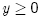under an inertial strip foundation with total mass per unit length, material density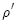, heightand widthas shown in Fig. 1. No temperature effect is considered for the problem. The foundation is subjected to a time-harmonic uniform force denoted by

whereandare the angular frequency and the Heaviside function, respectively.

Therefore the surface of the half-space is under the action of tractions for which

whereis the traction acting on the surface of the half-space and should be obtained considering the inertial effects of the strip foundation. Variation ofis assumed to be negligible on the width of the strip foundation and is assumed to be in the formis a constant which should be obtained regarding the inertial effects of the strip foundation. On the other hand, the solution of the problem should be obtained such that the stresses and the displacements vanish asapproaches to the infinity.

3. Solution

Navier's equation of motion for a homogeneous, isotropic and elastic medium without body forces is

whereandrepresent the density of the half-space's material and displacement vector, respectively.and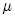are Lame's elastic constants for plane strain condition and are expressed as

whereandare elastic modulus and Poisson's ratio, respectively. Taking note of the harmonic time variation of the displacementsin the 2D case leads to

Consider the vector identity

and let

whereand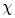are scalar and vector displacement potential functions, respectively. Hence equation in the 2D case will change to system of Poisson-type partial differential equations

whereis the third component of the vector displacement potential. Also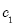andindicate the propagation velocity for longitudinal and torsional waves, respectively. Employing the Hooke's Law for an elastic body yields

Fourier integral transformation for the displacement potential functions can be expressed as

Taking Fourier transformation of equations and and considering the boundary conditions for infinite distances from the surface imply

whereandare functions to be obtained from the surface boundary conditions. Employing surface boundary conditions of equation (2), equations and in the transformed form yields the system of equations

from which

and

Displacement potential functions can be used to obtain the transformed displacement and stresses in the 2D case as

where:

3.1. Inertial effects

Dynamic response of a half-space under a foundation subjected to time-varying forces has been investigated in many research works. The problem considered in this study alters to a classical one when the inertial effects of the massive foundation are neglected. Therefore, this section is to propose convenient comparative parameters to illustrate the inertial effects which have been considered rarely in the previous works. These parameters would represent the differences between the results of the problem with and without considering the inertial effects.

The interaction loadbetween the strip foundation and the half-space from the prescribed time-harmonic loadcan be obtained considering the Newton's second law for the strip foundation

Because of higher rigidity of the foundation's material with respect to the rigidity of the material of the half-space, and the continuous contact between the foundation and the half-space, it is convenient to assume

For a strip foundation, the variation of the displacement under the width of foundation is negligible. Thus from equation , the vertical component of the surface displacement field is

where

The integral of equation can be obtained by replacing the integration along the real axis by integrals around the branch cuts, in addition to the contributions from the poles. This complicated procedure which is comparable with the procedure done by Lamb  yields the final results as

where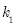has been chosen as the normalizing factor for all length parameters. Then,andis the positive real root ofwith

Assume thatbe the coefficient of the total load

Therefore the inertial load in equation will produce static and dynamic influences. Henceforth the effects of the second term which produces the static displacements are ignored for the sake of simplicity. Using equations and gives rise to:

where

and

Hereis a dimensionless parameter.andindicate the coefficient of the inertia effect and the phase angle, respectively. Dehestani et al  studied the inertial effect of a moving object on the dynamic response of beams by introducing a coefficient of inertia effect,. This coefficient is the ratio of the dynamic response for loads with inertial effects to the same loads without the inertial effects. The phase angleis another representative factor for the inertial effects of the foundation.

3.2. Steepest descent approximation

Due to the fact that the direct inversion of the transformed stresses and displacements is a rigorous procedure, steepest descent approximation has been considered. Now, a brief conceptual description of the steepest descent method will be presented from the book by Achenbach . Consider the integral

where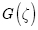andare analytical functions on the path of integration. Analytical functionin whichcan be expanded in complex form

where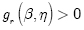.

For large, a small variation ofdue to a small change ofresults in swift fluctuations of the trigonometric functions. Ifis constant on the path of integration, the swift fluctuations will be disappeared and thenwill be the most rapidly varying part of the integrand. In this case, the main contribution to the integral in equation will arise from the neighborhood of the point, whereis smallest. It can be shown that the path throughdefined byis the path along whichvaries most rapidly, so that the requirement of concentrating the largest values ofto the shortest possible segment of the integration path is optimized along the path on which. The idea of the steepest descent approximation thus is to deform the path of integration in the-plane into a contour on whichand which passes through the point, whereis stationary.

Employing the steepest descent method yields:

where the parameters,and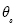can be obtained form

Now consider the integral

where

From equations , and we have

Hence from equation

Eventually, the displacements and the stresses in the 2D case take the form:

where:

4. Numerical example

As an illustration of the proposed solution approach, consider a half-space with mechanical properties:

Assume that the loading conditions on a foundation with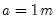be

Then, the parameters of inertial effects can be obtained from and as:

Eventually, variations of the real and imaginary parts of the displacements and the stresses are obtained and are shown in Fig. 2 and Fig. 3.

Figures show a fluctuating behavior for displacements and stresses with respect to the distances from the foundation. Values of the real parts and the imaginary parts in displacements and stresses are in the same range indicating a phase-type difference between them.

In fact the main reason for this type of behavior is related to the definition of the prescribed load. Harmonic force consists of two sinusoidal and co-sinusoidal parts which are different in their phase angle.

As shown in the figures, the stresses and displacements tend to vanish as the distance to the inertial strip foundation increases. Also with increase in the distance the fluctuating behavior is reduced.

Most of the physical time-dependent functions can be approximated by a Fourier complex series expansion on an arbitrary domain. Hence results obtained for time-harmonic loads in this paper can be used to obtain the approximate stresses and displacements for arbitrary time-dependent loadings in specified time domains. To this aim, any kind of time-varying force on the foundation should be expanded by a complex Fourier expansion in its duration. By evaluating the coefficients of the force function and the results of this paper, the final displacements and stresses can be found by superposition method.

It should be noted that the solution procedure for obtaining the far field behavior cannot be used for investigating near-field behavior. This is due to the fact that the convergence of the integral which was obtained by steepest descent method cannot be warranted.

5. Summary and concluding remarks

Dynamic behavior of a homogeneous, isotropic elastic half-space under an inertial strip foundation subjected to a time-harmonic force was investigated in this study. To this aim, Navier's equation of motion for the half-space was altered to system of Poisson-type partial differential equation. Fourier integral transformation was employed to solve the system of equation considering the boundary conditions. Influences of the inertia of the strip foundation were considered in obtaining the surface boundary conditions. Steepest descent approximation was employed to obtain the two-dimensional displacements and stresses in the 2D case. Values of the real and imaginary parts of the stresses and the displacements were obtained for various horizontal and vertical distances in a numerical example. Investigation of exact dynamic behavior of half-space subjected to an inertial foundation can be considered for future work.

In revised form 22 May 2012

•  The eld and radiation impedance of mechanical radiators on the free surface of a semi-infinite isotropic solid, volume 223 of Series A. Proceedings of the Royal Society of London, 1954.
•  J.D. Achenbach. Wave propagation in elastic solids North-Holland Amsterdam, The Netherlands, 1973.
•  Mita Akira and J. Enrique Luco. Dynamic response of a square foundation embedded in an elastic half-space. Soil Dynamics and Earthquake Engineering, 8(2):54-67, 1989.
•  L. Andersen, Nielsen S.R.K., and S. Krenk. Numerical methods for analysis of structure and ground vibration from moving loads. Computers and Structures, 85:43-58, 2007.
•  Kaczyski Andrzej and J. Matysiak Stanisaw. On 3d punch problems for a periodic two-layered elastic half-space. Journal of Theoretical and Applied Mechanics, 39(3):523-538, 2001.
•  T. Balendra and A.C. Heidebrecht. Harmonic response of buildings interacting with soil. Journal of Sound and Vibration, 117(2):351-361, 1987.
•  Bilello Cristiano, A. Bergman Lawerence, and Kuchma Daniel. Experimental investigation of a small-scale bridge model under a moving mass. ASCE Journal of Structural Engineering, 130(5):799-804, 2004.
•  M. Dehestani, M. Mofid, and A. Vafai. Investigation of critical in uential speed for moving mass problems on beams. Applied Mathematical Modelling, 33:3885-3895, 2009.
•  M. Dehestani, A. Vafai, and M. Mofid. Steady-state stresses in a half-space due to moving wheel-type loads with nite contact patch. Scientia Iranica, Transactions A: Civil Engineering, 17(5):387-395, 2010.
•  M. Dehestani, A. Vafai, and M. Mofid. Stresses in thin-walled beams subjected to a traversing mass under a pulsating force,. Journal of Mechanical Engineering Science, 224 (11):2363-2372, 2010.
•  M. Dehestani, A. Vafai, M. Mofid, and G. Ahmadi. On the dynamic response of a half-space subjected to a moving mass. Mathematics and Mechanics of Solids, 17(4):393-412, 2012.
•  L. Karabalis Dimitris and D. Huang Chu-Feng. Inertial soil-foundation interaction by a direct time domain bem. Mathematics and Computer Modelling, 15(3-5):215-228, 1991.
•  L. Fryba. Vibrations of Solids and Structures under Moving Loads Thomas Telford Ltd, London, 1999.
•  P. Galvin and J. Dominguez. High-speed train-induced ground motion and interaction with structures. Journal of Sound and Vibration, 307:755-777, 2007.
•  F. Guan, I.D. Moore, and C.C. Spyrakos. Two dimensional transient fundamental solution due to suddenly applied load in a half-space. Soil Dynamics and Earthquake Engineering, 17:269-277, 1998.
•  S. Guenfoud, S.V. Bosakov, and D.F. Laefer. Dynamic analysis of a beam resting on an elastic half-space with inertial properties. Soil Dynamics and Earthquake Engineering, 29:1198-1207, 2009.
•  Z. Hryniewicz. Dynamic response of a rigid strip on an elastic half-space, 1981.
•  P. Koziol and C. Mares. Wavelet approach for vibration analysis of fast moving load on a viscoelastic medium. Shock and Vibration, 17(4-5):461-472, 2010.
•  P. Koziol, C. Mares, and I. Esat. Wavelet approach to vibratory analysis of surface due to a load moving in the layer. International Journal of Solids and Structures, 45:2140-2159, 2008.
•  Horace Lamb. On the propagation of tremors over the surface of an elastic solid, volume 203 of Series A. Philosophical Transactions of the Royal Society of London, Londan, 1904.
•  Guangyu Liu and Kaixin Liu. Exact solution for a two-dimensional lambs problem due to a strip impulse loading. 20(3):258-265, 2007.
•  Pawlik Marcin and Rogowski Bogdan. Adhesive contact problem for transversely isotropic elastic half-space. Journal of Theoretical and Applied Mechanics, 41(1):89-105, 2003.
•  Rades Mircea. Dynamic analysis of an inertial foundation model, international, 1972.
•  J. Qian and D.E. Beskos. Harmonic wave response of two 3-d rigid surface foundations. Soil Dynamics and Earthquake Engineering, 15:95-110, 1996.
•  Paolucci Roberto and Spinelli Davide. Ground motion induced by train passage,. Journal of Engineering Mechanics,, 132(2):201-210, 2006.
•  M.R. Shadnam, M. Mofid, and J.E. Akin. On the dynamic response of rectangular plate, with moving mass,. Thin-Walled Structures, 39:797-806, 2000.
•  C.M. Wang, Y.K. Chow, and Y.C. How. Analysis of rectangular thick rafts on an elastic half-space. Computers and Geotechnics, 28:161-184, 2001.
• *
Author email:
• # Publication Dates

• Publication in this collection
01 Mar 2013
• Date of issue
May 2013